# Titanium Depletion Including Recycling

The graph below shows the titanium extraction data for the world and two Verhulst function fits to the data in order to extrapolate into the future.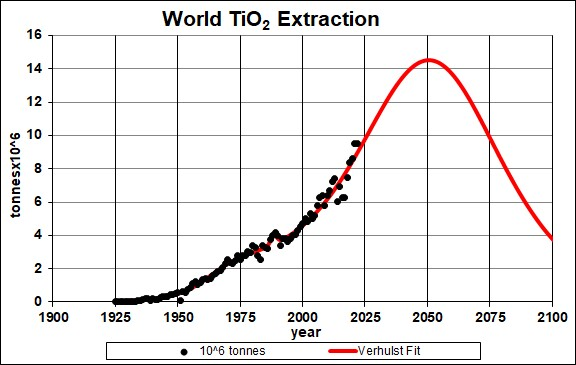Tintanium (TiO2) extraction rate for the world and a Verhulst function fit to the data.

The curve is obtained by restricting the total extraction to the amount already extracted plus the estimated reserve of 1200x106 tonnes.

It appears that the recent rapid rise in extraction rate is unsustainable for more that a few decades or so from now.

Taking an average extraction curve of the two fits, the crossover point at ~2050 when the amount extracted is equal to the amount left to be extracted is shown here: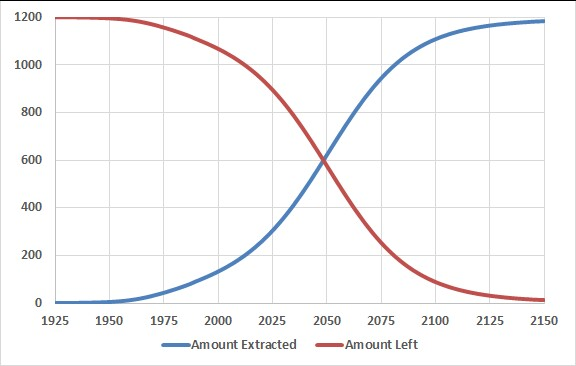## Recycling

Assume that:

• The titanium extraction curve is the average of the two curves given above.
• Recycling of titanium follows a hyperbolic tangent curve from 50% to 84% recycling with a break point of year 1950 and width 10 years, beginning in year 1970.
• The recycling is delayed by a Gaussian curve peaking at a delay of 15 years and a width of 10 years.

The effective titanium available for making items after the first ten recycling cycles is shown in the following graph, along with the effective titanium available for each cycle: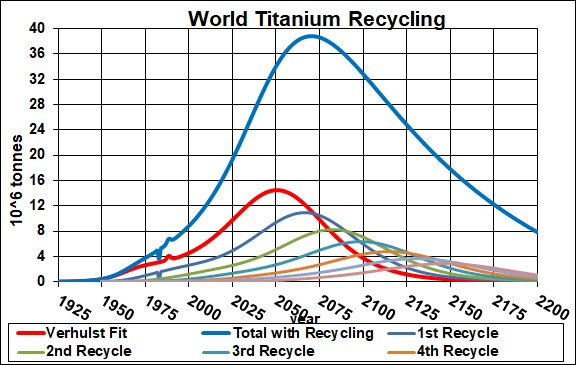The equation for a recyling cycle is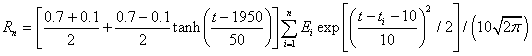,

where Ei is the amount available from the previous cycle. Here is an example of the Excel coding:

{=((\$J\$2+\$I\$2)/2+((\$J\$2-\$I\$2)/2)*TANH((A27-\$K\$2)/\$L\$2))*SUM(\$I\$27:I27*(EXP(-1*((A27-\$A\$27:A27-\$N\$2)/\$O\$2)^2/2))/\$O\$2/SQRT(2*PI()))} (The curly bracket surrounding the term makes it into an array; it must be entered by holding down the SHIFT & CTRL keys while pressing the ENTER key.)

Thus, under the assumptions given above, the effective amount of titanium available for making items peaks at about year 2040 and falls off rapidly after that. Humans will have taken concentrated titanium deposits and scattered them across the surface of the earth.

The Excel spreadsheet is set up to make it easy to calculate with different recycling assumptions.

## Titanium Per Capita

Dividing the copper recycling curve above by the projected world population (asymptote ~1010), one gets: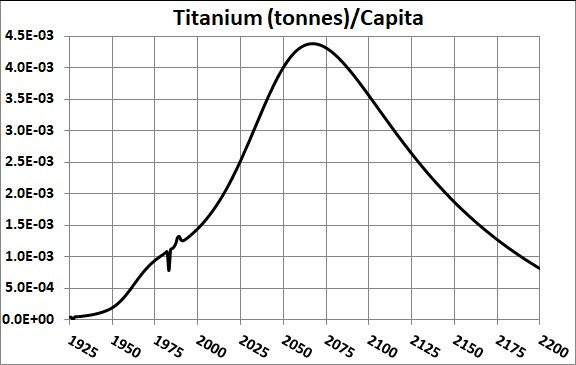Minerals Depletion